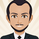2336 görüntülenme
Adaptive Moving Average indicator script. This indicator was originally developed by Vitali Apirine (Stocks & Commodities V.36:5: Adaptive Moving Averages).
Sürüm Notları: Refactored
Sürüm Notları:
A list of Free indicators:
https://bit.ly/2S7EPuN

A list of Paid indicators:
https://bit.ly/33MA81f

Earn \$30:

Toss a coin to your witcher:
PayPal: https://www.paypal.me/alexeverget

## Yorumlar"

inputs:
Price( numericseries ),
EffRatioLength( numericsimple ),
FastAvgLength( numericsimple ), { this input assumed to be a constant >= 1 }
SlowAvgLength( numericsimple ) ; { this input assumed to be a constant >= 1 }

{ Eff = Efficiency }

variables:
NetChg( 0 ),
TotChg( 0 ),
EffRatio( 0 ),
ScaledSFSqr( 0 ),
SlowAvgSF( 2 / ( SlowAvgLength + 1 ) ),
FastAvgSF( 2 / ( FastAvgLength + 1 ) ),
SFDiff( FastAvgSF - SlowAvgSF ) ,
pds(0),
MLTP(0),
SSC(0);

pds = EffRatioLength + 1;
MLTP = AbsValue(Price - Lowest(low, pds)) - (highest(high, pds) - price) / (highest(high, pds) - lowest(low, pds));
SSC = MLTP * (FastAvgSF - SlowAvgSF) + SlowAvgSF;

"

This is what I have so far, when plugged into Tradestation along with an indicator to plot it the AMA comes out significantly different than the one on my chart from tradingview. Thanks in advance.
Cevap GönderHi,
Function: _AMA

// TASC APR 2018
// _AMA
// Function
// Vitali Apirine

inputs:
Periods( numericsimple ),
FastAvgLength( numericsimple ),
SlowAvgLength( numericsimple ) ;

variables:
PDS( Periods + 1 ),
FastSC( 2 / ( FastAvgLength + 1 ) ),
SlowSC( 2 / ( SlowAvgLength + 1 ) ),
SSC( 0 ),
CST( 0 ),
MLTP( 0 );

MLTP = AbsValue( ( Close - Lowest( Low, PDS ) )
- ( Highest( High, PDS ) - Close ))
/ ( Highest( High, PDS ) - Lowest( Low, PDS ) ) ;

SSC = MLTP * ( FastSC - SlowSC ) + SlowSC ;

CST = Square( SSC ) ;

if CurrentBar = 1 then
_AMA = Close + CST * ( Close - Close )
else
_AMA = _AMA + CST * ( Close - _AMA ) ;

--------------------------------------------------------------------

// TASC APR 2018
// Indicator
// Vitali Apirine

inputs:
Periods( 10 ),
FastAvgLength( 2 ),
SlowAvgLength( 30 ) ;

variables:
AMA( 0 ),
KAMA( 0 ) ;

AMA = _AMA( Periods, FastAvgLength,
SlowAvgLength ) ;
FastAvgLength, SlowAvgLength ) ;

Plot1( AMA, "AMA", Cyan ) ;
Plot2( KAMA, "KAMA", Magenta ) ;
Cevap Göndereverget
@everget, Thank you so much! I truly appreciate it and the speedy help.
Cevap Gönder